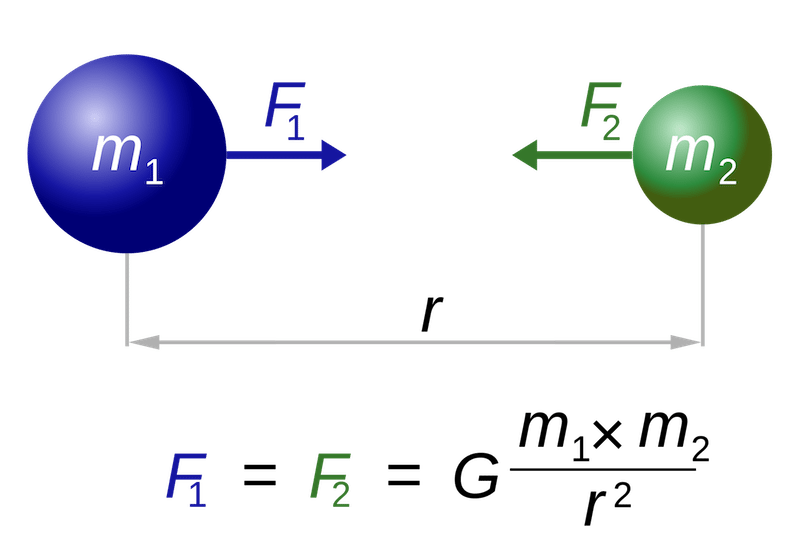# Theory of Universal Gravitation

04.11.2015

Episode #3 of the course “Ideas that changed the world”

The theory of universal gravitation explains the relationships of force between objects in the universe. Before Sir Isaac Newton promoted this concept, “gravity” had not yet been invented. However, after he published Principia in 1687, scientific understanding of the nature of the universe would never be the same. Newton was the most important scientist of the 17th century, and his theories formed the basics of physics study for the next 250 years. He claimed that the amount of force exerted by one body on another is in direct proportion to the two objects’ size and distance. He measured this force and called it “gravity.”

By studying astronomy, physics, and mathematics, Newton made new discoveries about light and the movement of celestial objects in space. In particular, Newton strove to explain the movement and physical relationship of the moon to the earth, and it is likely from this that he developed his theory of gravity. Newton asserted that this force could be applied to any objects and it would still function in predictable ways. He formulated rules to predict how bodies that are spinning would act, in addition to bodies that were resting or moving in one direction or another.According to the law of universal gravitation, the attractive force (F) between two bodies is directly proportional to the product of their masses (m1 and m2), and inversely proportional to the square of the distance, r, (inverse-square law) between them. The constant of proportionality, G, is the gravitational constant.

The remarkable thing about Newton’s theory of gravity was that it did not simply apply to large or celestial bodies, but could be used to explain a relationship of force between any two physical bodies. While astronomers studied the stars, other physicists turned to earth.

This concept, and the formulas and equations Newton calculated to accompany it, changed how scientists understood the nature of the universe. Not only did Newton’s explanations advance the knowledge of astrophysics and the relationships of celestial bodies, but naturalist scientists could make advances about the nature of reality on earth based on Newtonian principles. Although unintended by Newton, gravity became so commonly understood that it is referenced in children’s movies and songs.

Quotes

“If I have seen further than others, it is by standing upon the shoulders of giants.” by Isaac Newton

“What is there in places empty of matter? and Whence is it that the sun and planets gravitate toward one another without dense matter between them? Whence is it that Nature doth nothing in vain?” by Isaac Newton

“I have not been able to discover the cause of those properties of gravity from phenomena, and I frame no hypotheses.” by Isaac Newton

“At the end of the [19th] century no extension or analogue of the Newtonian gravitation formula has been generally accepted, and it still stands there as almost the only firmly established mathematical relation, expressive of a property of all matter, to which the progress of more than two centuries has added nothing, from which it has taken nothing away.” by John Theodore Merz

“A genius is someone who discovers that the stone that falls and the moon that doesn’t fall represent one and the same phenomenon.” by Ernesto Sábato

Recommended books

“The Principia : Mathematical Principles of Natural Philosophy” by Isaac Newton“Isaac Newton” by James GleickShare with friends